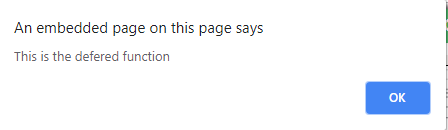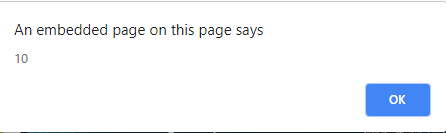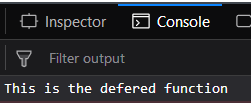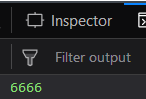# Underscore.js _.defer() Function

• Last Updated : 25 Nov, 2021

The Underscore.js is a JavaScript library that provides a lot of useful functions like the map, filter, invoke etc even without using any built-in objects.
The _.defer() function is used to invoke/call a function until the current call stack is cleared. Its major advantage is that it performs expensive computations, calculations or HTML in chunks without blocking the UI threads from updating. It has similar functioning to setTimeOut() function with a delay of 0. The function which is passed to this function will be invoked first.
A call stack is a mechanism for an interpreter like the JavaScript interpreter, to keep a track of its place in a script that calls multiple functions.
Syntax:

`_.defer(function, *arguments)`

Parameters:
It takes two arguments:

• The function
• The arguments (optional)

Return value:
It does not return any value rather it performs the function passed.

1. Passing alert() function to the _.defer() function:
The _.defer() function first checks the current calling stack. If it is cleared then the alert() function passed will be performed and the string passed to it which is “This is the deferred function” will be displayed. But if the current calling stack is not cleared and has some other jobs to be performed then the alert() function will not be called immediately rather it needs to wait until the stack becomes empty.
Examples:

## html

 `<``html``> ` ` `  `<``head``> ` `    ``<``script` `src``= ` `"https://cdnjs.cloudflare.com/ajax/libs/underscore.js/1.9.1/underscore-min.js"``> ` `  `` ` ` ` ` `  `<``body``> ` `    ``<``script` `type``=``"text/javascript"``> ` `        ``_.defer(function() { ` `            ``alert('This is the deferred function'); ` `        ``}); ` `    `` ` ` ` ` `  ` `

1. Output:1.
2. Performing addition using _.defer() function:
We can perform all kinds of mathematical operations using the _.defer() function. Like here we are performing addition of 7 and 3 and then alerting it. The same procedure will be followed. First, the current calling stack will be checked. If it is not empty then no other function will be called. But if it is all cleared then the alert function will be called displaying the addition of 7 and 3 which is 10.
Example:

## HTML

 `<``html``> ` ` `  `<``head``> ` `    ``<``script` `src``= ` `"https://cdnjs.cloudflare.com/ajax/libs/underscore.js/1.9.1/underscore-min.js"``> ` `  `` ` ` ` ` `  `<``body``> ` `    ``<``script` `type``=``"text/javascript"``> ` `        ``_.defer(function() { ` `            ``alert(7 + 3); ` `        ``}); ` `    `` ` ` ` ` `  ` `

1. Output:1. Passing console.log() function to the _.defer() function:
We can even use other functions like the console.log() function. The _.defer() function will check whether the calling stack is empty or not. If it is not empty then the console.log() function will not be called. Otherwise, “This is the deferred function” will be displayed on the console.
Example:

## html

 `<``html``> ` ` `  `<``head``> ` `    ``<``script` `src``= ` `"https://cdnjs.cloudflare.com/ajax/libs/underscore.js/1.9.1/underscore-min.js"``> ` `  `` ` ` ` ` `  `<``body``> ` `    ``<``script` `type``=``"text/javascript"``> ` `        ``_.defer(function() { ` `            ``console.log('This is the deferred function'); ` `        ``}); ` `    `` ` ` ` ` `  ` `

1. Output:1.
2. Performing addition operation to the _.defer() function:
We can even perform addition operation to the console.log() function. Like here we are performing addition of 1000 and 5666. If the calling stack is empty then, “6666” (1000 + 5666) will be displayed otherwise no function will be called.
Example:

## html

 `<``html``> ` ` `  `<``head``> ` `    ``<``script` `src``= ` `"https://cdnjs.cloudflare.com/ajax/libs/underscore.js/1.9.1/underscore-min.js"``> ` `  `` ` ` ` ` `  `<``body``> ` `    ``<``script` `type``=``"text/javascript"``> ` `        ``_.defer(function() { ` `            ``console.log(1000 + 5666); ` `        ``}); ` `    `` ` ` ` ` `  ` `

1. Output:1.

NOTE:
These commands will not work in Google console or in firefox as for these additional files need to be added which they didn’t have added.
 `<``script` `type``=``"text/javascript"` `src` `= ` `"https://cdnjs.cloudflare.com/ajax/libs/underscore.js/1.9.1/underscore-min.js"``> ` ` `